# 在React中使用Plotly来构建动态图表

Plotly是一个免费的开源数据可视化框架，它提供了多种绘图类型，如线形图、散点图、直方图、考克斯图等等。

## 前提条件

``` npm install react-plotly.js plotly.js npm i @chakra-ui/react @emotion/react@^11 @emotion/styled@^11 framer-motion@^4

```

## Plotly如何工作

```

```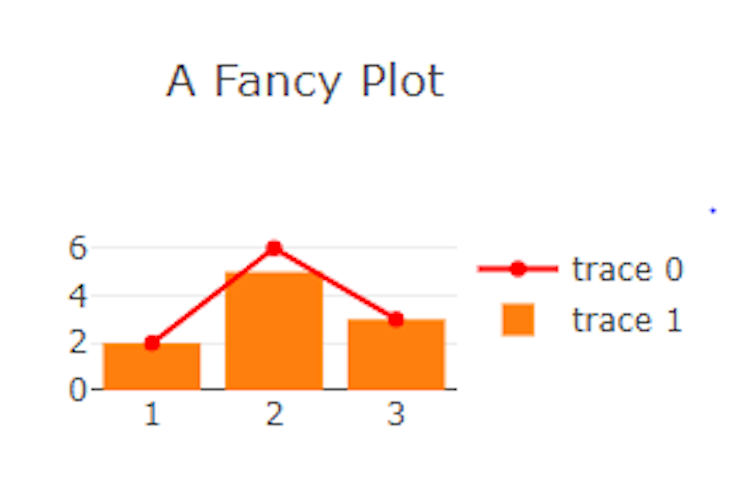## Plotly图形和图表的不同类型

Plotly超越了简单的柱状图和散点图。我们将回顾我们可以用Plotly实现的其他图表以及如何在React中使用它们

### 分组条形图

``` const GroupedBarChart = (props) =>{ var plot1 = { x: ["Microwave", "Washing Machine", "Tv", "Vacuum Cleaner", "Hair Dryer"], y: [4, 5, 6, 1, 4], name: "2016", type: "bar", };

var plot2 = { x: ["Microwave", "Washing Machine", "Tv", "Vacuum Cleaner", "Hair Dryer"], y: [12, 3, 5, 6, 2], name: "2017", type: "bar", };

var data = [plot1, plot2]; return( ) }

```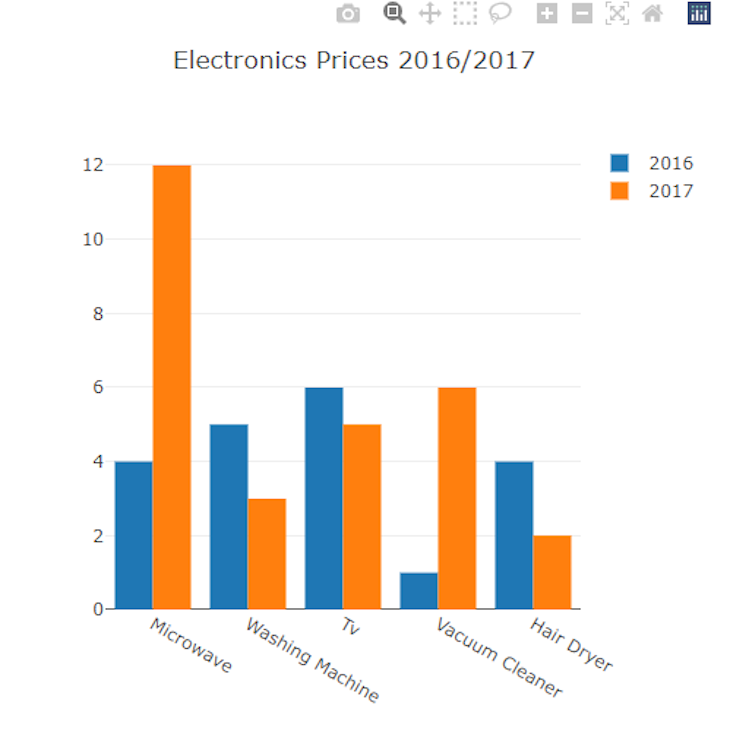### 饼图

``` const PieChart = (props) =>{ var data = [ { values: [112, 454, 65, 544], labels: ["Blue", "Red", "Yellow", "Orange"], type: "pie", }, ];

``````return(
<Plot
data={data}
layout={ {width: 500, height: 500, title: 'Favourite Colours In A Class'} } />
)
``````

}

```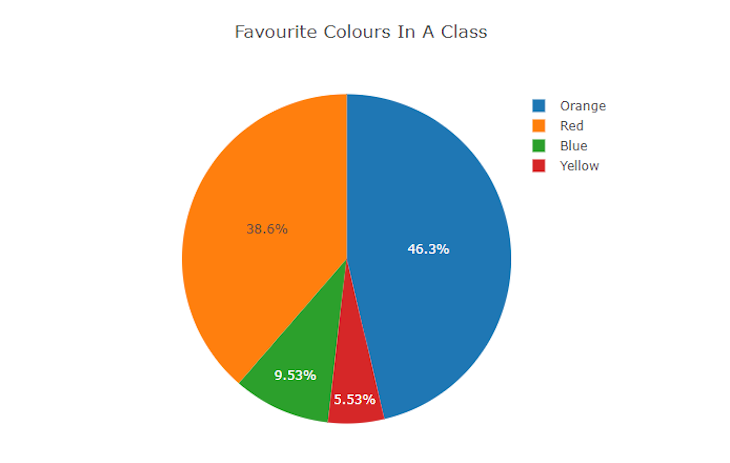### 面积图

``` const AreaChart = (props) =>{ var data = [ { x: [1, 2, 3, 4], y: [0, 2, 3, 5], fill: 'tozeroy', type: 'scatter', name: 'Vendor' }, { x: [1, 2, 3, 4], y: [3, 5, 1, 7], fill: 'tonexty', type: 'scatter', name: 'Provider' } ];

``````return(
<Plot
data={data}
layout={ {width: 500, height: 500, title: 'Area Chart'} } />
)
``````

}

```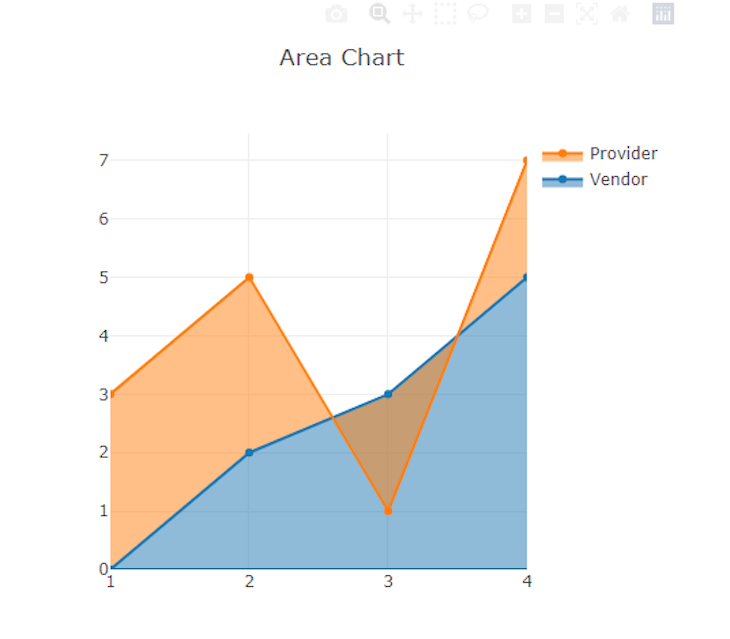### 表格

``` const Table= (props) =>{ const values = [ ["Books", "Clothes", "Medicals"], ["\$22", "\$190", "\$516"], ]; const headers = [[" Item "], [" Expenditure "]]; const data = [ { type: "table", header: { values: headers, align: "center", }, cells: { values: values, align: "center", }, }, ];

``````return(
<Plot
data={data}
layout={ {width: 500, height: 500, title: 'Table'} } />
)
``````

}

```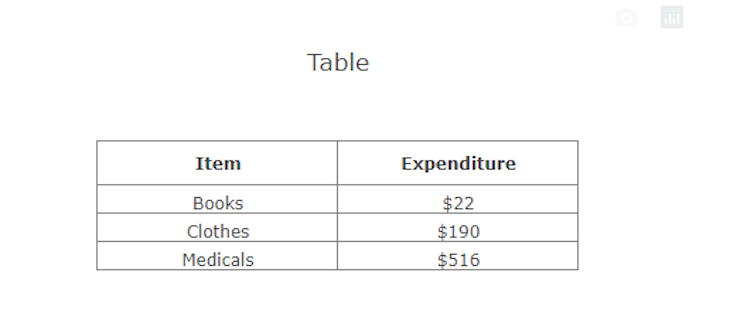``` const Table= (props) =>{ const values = [ ["Books", "Clothes", "Medicals"], ["\$22", "\$190", "\$516"], ]; const headers = [[" Item "], [" Expenditure "]]; const data = [ { type: "table", header: { values: headers, align: "center", fill: { color: "#2849cc" }, font: { color: "white", size: 20 }, }, cells: { values: values, align: "center", font: { family: "Ubuntu" }, }, }, ];

``````return(
<Plot
data={data}
layout={ {width: 500, height: 500, title: 'Table'} } />
)
``````

}

```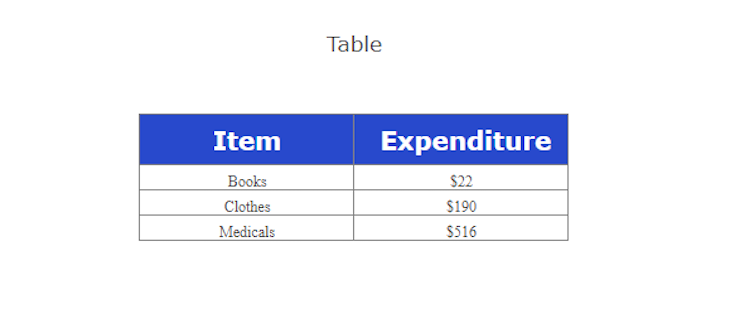## 用Plotly创建动态图表

``` import Plot from 'react-plotly.js'; import React, { useState } from "react" import { HStack,Input, Text } from "@chakra-ui/react"

const GraphCard = (props) =>{

const [ scatter, setScatter ] = useState( { x: [1,2,3], y: [2,5,3], type: 'scatter', mode: 'lines+markers', marker: {color: 'red'}, } ); const [ data, setData ] = useState([scatter]);

const handleChange = (i,j,e) => { const newScatter = {...scatter}; newScatter[i][j]=parseInt(e.target.value); setScatter(newScatter); setData([scatter]); }

``````return(
<>
<Plot data={[{
x: \[data[0\]["x"]\,data[0\]["x"]\,data[0\]["x"]],
y: \[data[0\]["y"]\,data[0\]["y"]\,data[0\]["y"]],
type: 'scatter',
mode: 'lines+markers',
marker: {color: 'red'},
}]} layout={ {width: 500, height: 500, title: 'A Dynamic Fancy Plot'} } />
<Text fontSize="md">X1</Text>
<Input type="number" placeholder={data\[0\]["x"]} onChange={(e) => handleChange("x",0,e)}/>
<Text fontSize="md">X2</Text>
<Input type="number" placeholder={data\[0\]["x"]} onChange={(e) => handleChange("x",1,e)}/>
<Text fontSize="md">X3</Text>
<Input type="number" placeholder={data\[0\]["x"]} onChange={(e) => handleChange("x",2,e)}/>
<Text fontSize="md">Y1</Text>
<Input type="number" placeholder={data\[0\]["y"]} onChange={(e) => handleChange("y",0,e)}/>
<Text fontSize="md">Y2</Text>
<Input type="number" placeholder={data\[0\]["x"]} onChange={(e) => handleChange("y",1,e)}/>
<Text fontSize="md">Y3</Text>
<Input type="number" placeholder={data\[0\]["x"]} onChange={(e) => handleChange("y",2,e)}/>
</HStack>

)
``````

}

export default GraphCard;

```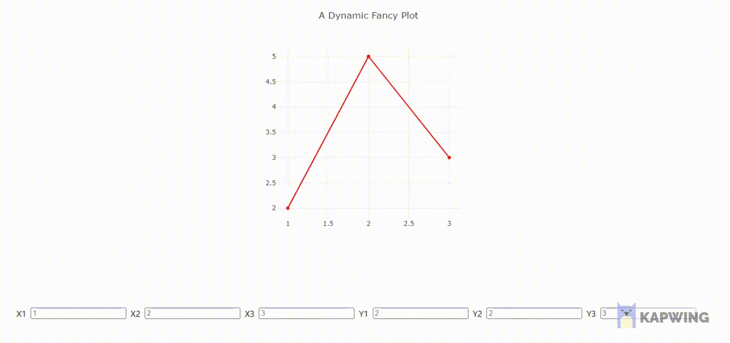## 用Plotly定制图表

### 标注轴

```

```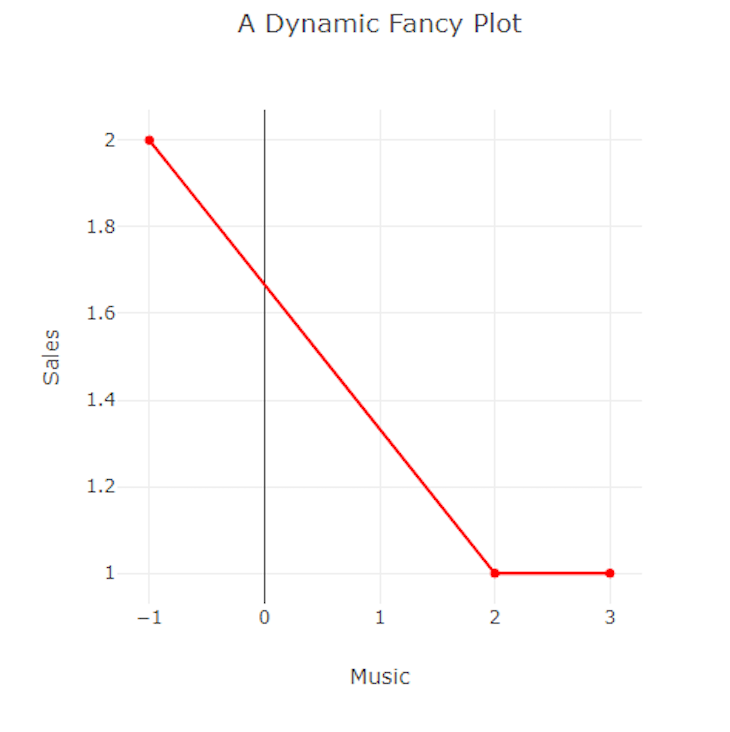### 滚动到缩放

```

```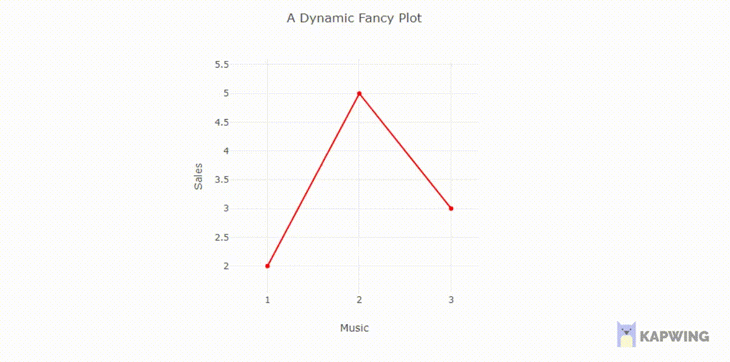### 添加图例名称

```

```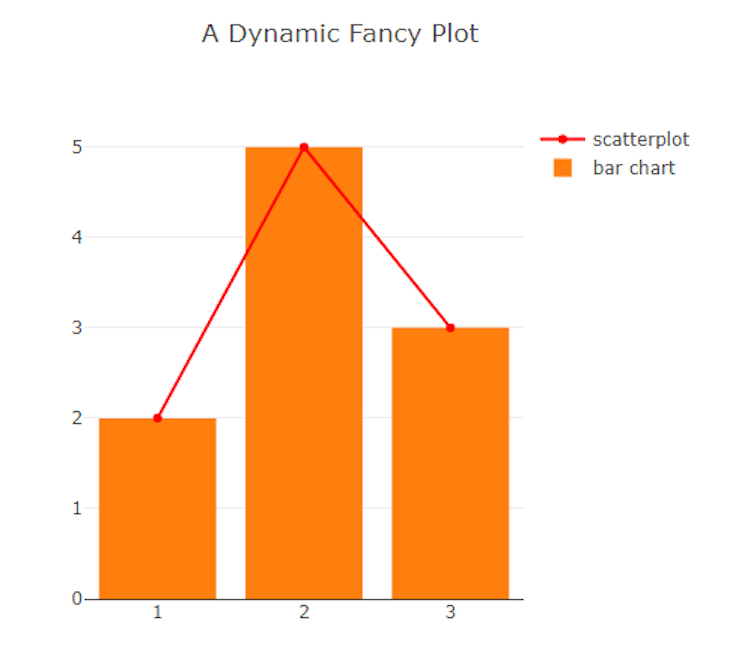## 总结

The postUsing Plotly in React to build dynamic charts and graphsappeared first onLogRocket Blog.